Next: Properties of average Up: Useful Properties Previous: Range Analysis

### Relationship between cons' and average

The cons' operation is a primitive operation on lists and streams. Operations on exact real numbers, however, are defined in terms of mathematical operations. Fortunately the semantics of the stream of digits representation of reals leads to a useful relationship between cons' and average which simplifies later definitions.

We define average as one would expect, using the symbol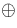so that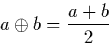Now, suppose we wish to compute the average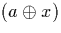of the digit a and the stream x. It is useful to observe that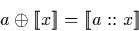because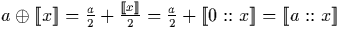. Hence the result of averaging a digit and a stream may be performed by generating a new stream using the cons' operation to attach the digit at the head of the original stream.

Martin Escardo
5/11/2000